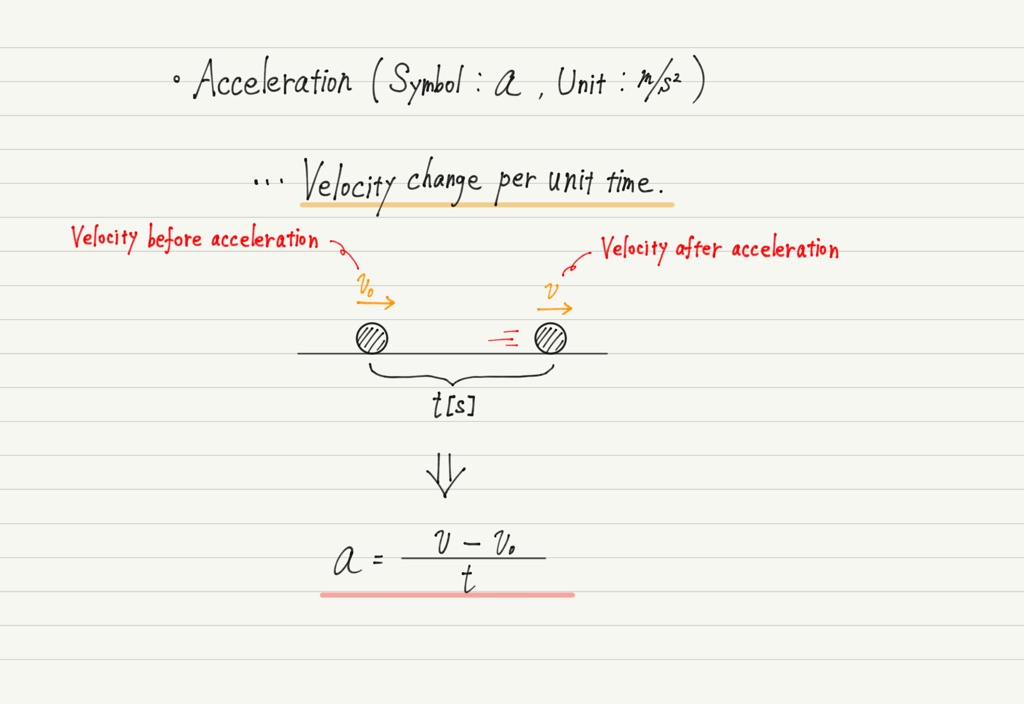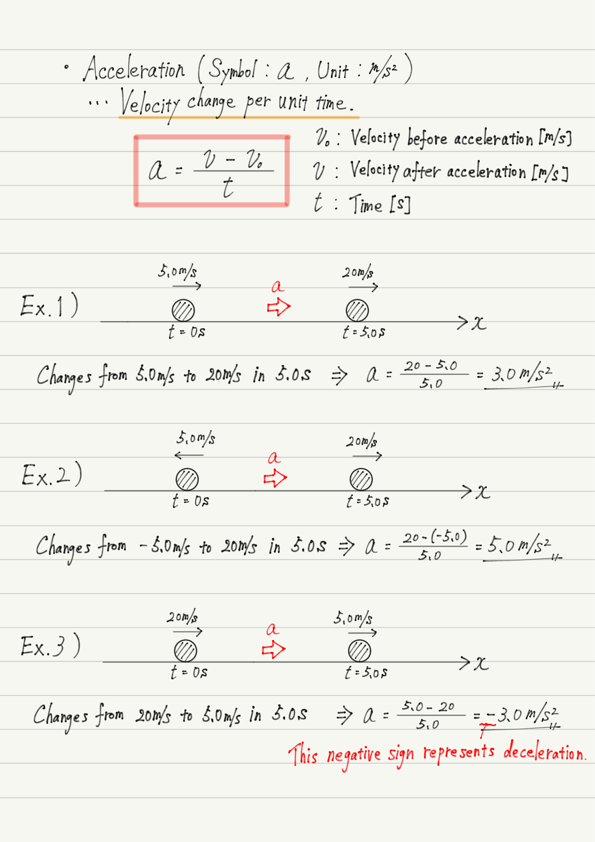# Acceleration

We will study the first difficulty in mechanics, acceleration motion.

So far, the only specific motion we have learned about is uniform linear motion.
Uniform linear motion is not very interesting because there is no change in speed or direction.
Therefore, let’s go one step further from uniform linear motion and deal first with motion with varying velocity.

## How to express changes in velocity

Acceleration represents the change in velocity. However, it is not simply a question of how much the speed has increased.

If an object moving at 10 m/s accelerates to 50 m/s, the change in velocity is “an increase of 40 m/s.”
However, whether this is a 1-second event or a 30-minute event makes all the difference.

For the same change in velocity, a change in a shorter period of time can be said to be “more accelerated”.

Based on the above, let us define “Acceleration = Change in velocity per unit time”.Specific calculations of acceleration are described in the summary of this lecture.

## Points to note

There are two things to note about acceleration.

The first point is the word in the definition given above. Read it carefully.
Acceleration is the “velocity” change per unit time. It is not a “speed” change!

If you are unclear about the difference between speed and velocity, check the previous article. If you do not know this, you will not be able to calculate acceleration correctly.

https://www.yukimura-physics.com/entry/dyn-f03

And the second point. Acceleration, like velocity, has a direction.
The direction of acceleration, like velocity and displacement, is represented by a plus or minus sign, but special care must be taken when the acceleration is negative.
Negative acceleration represents not only the phenomenon of “acceleration in the negative direction” but also “deceleration in the positive direction.

Be careful, because you need to judge from the context which meaning is being used.

## Summary of this lecture## Next Time

The next lecture will be a stepping stone. Three formulas for accelerated motion will make an appearance!

https://www.yukimura-physics.com/entry/dyn-f06

error: Content is protected !!